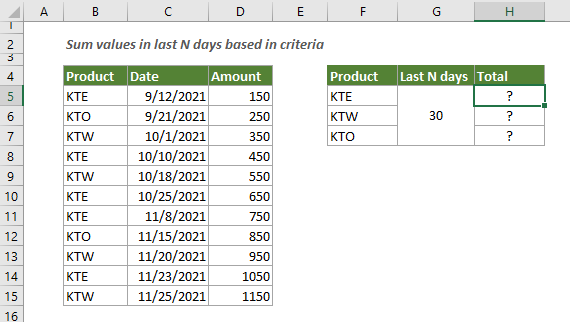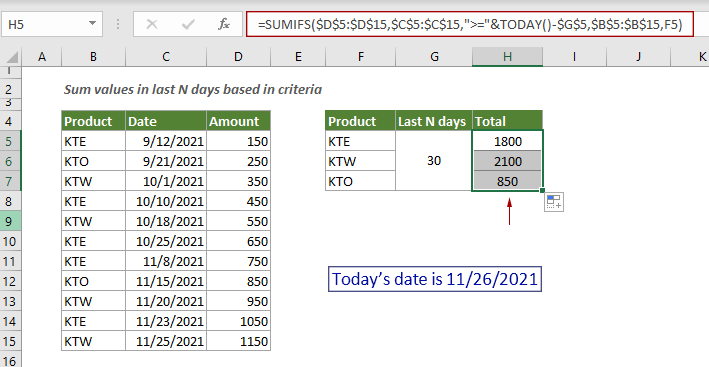## Sum values in last N days based on criteria in Excel

To sum values in last N days based on specific criteria in Excel, you can apply a formula based on the SUMIF and TODAY function.

#### How to sum values in last N days based on criteria in Excel?

As shown in the example below, there is a product sales table, to sum the amounts for each product sold in the last 30 days, you can follow the steps below.Generic Formulas

=SUMIFS(data_range,date_range,">="&TODAY()-N,criteria_range,criteria)

Arguments

Data_range: The range of cells you want to sum. In this example, it is D5:D15;
Date_range: The date range that you want evaluated by the last N days. It is C5:C15;
N: A number represents the last n days;
Criteria_range: The range of cells that you want evaluated by the criteria; It is B5:B15;
Criteria: The criterion defines which values in the Criteria_range will be summed.

How to use this formula?

Select a cell, copy or enter the formula below and press the Enter key to get the result. Select the result cell and then drag its AutoFill Handle down to get the results by other criteria.

=SUMIFS(\$D\$5:\$D\$15,\$C\$5:\$C\$15,">="&TODAY()-\$G\$5,\$B\$5:\$B\$15,F5)Explanation of the formula

=SUMIFS(\$D\$5:\$D\$15,\$C\$5:\$C\$15,">="&TODAY()-\$G\$5,\$B\$5:\$B\$15,F5)

\$C\$5:\$C\$15,">="&TODAY()-\$G\$5: Firstly, checks if each date in the date_range “\$C\$5:\$C\$15” is greater than or equal to the current date minus 30;
(C5:H10)*({FALSE;FALSE;TRUE;FALSE;FALSE;FALSE}): The TURE and FALSE are converted to 1 and 0. Each values in C5:H10 is multiplied by values in the array, after multiplying, you will get another array, here I show the array in the table below:
\$B\$5:\$B\$15,F5: Then checks if each cells in the criteria_range “\$B\$5:\$B\$15” is equals to the value in cell F5, “KTE”;
Then the SUMIFS function sums all amounts (in \$D\$5:\$D\$15) for the product “KTE” sold in the last 30 days.

#### Related functions

Excel SUMIFS function
The Excel SUMIFS function can help to sum cell values based on multiple criteria.

Excel TODAY function
The Excel TODAY function is used for returning the current system date in Excel.

#### Related formulas

Sum multiple columns if one criterion is met
This tutorial will guide you how to sum values in multiple columns based on the same criterion in Excel.

Sum the last N columns
To sum values in the last n columns, normally you may need to calculate the sum of each column and then add up the results to get the final sum. This tutorial provides a formula based on the INDEX function to help you easily get it done.

Sum top N values or top N values with criteria
This step-by-step tutorial demonstrates how to use the SUMPRODUCT function with the LARGE function to sum the top N values in a range and sum the top N values in a range with criteria in Excel.

Sum values based on column or both column and row
This tutorial provides different formulas based on the SUMPRODUCT function to help you sum values based on a column header, and sum values based on both column and row criteria.

### The Best Office Productivity Tools

#### Kutools for Excel - Helps You To Stand Out From Crowd

Would you like to complete your daily work quickly and perfectly? Kutools for Excel brings 300 powerful advanced features (Combine workbooks, sum by color, split cell contents, convert date, and so on...) and save 80% time for you.

• Designed for 1500 work scenarios, helps you solve 80% Excel problems.
• Reduce thousands of keyboard and mouse clicks every day, relieve your tired eyes and hands.
• Become an Excel expert in 3 minutes. No longer need to remember any painful formulas and VBA codes.
• 30-day unlimited free trial. 60-day money back guarantee. Free upgrade and support for 2 years.#### Office Tab - Enable Tabbed Reading and Editing in Microsoft Office (include Excel)

• One second to switch between dozens of open documents!
• Reduce hundreds of mouse clicks for you every day, say goodbye to mouse hand.
• Increases your productivity by 50% when viewing and editing multiple documents.
• Brings Efficient Tabs to Office (include Excel), Just Like Chrome, Firefox, And New Internet Explorer.